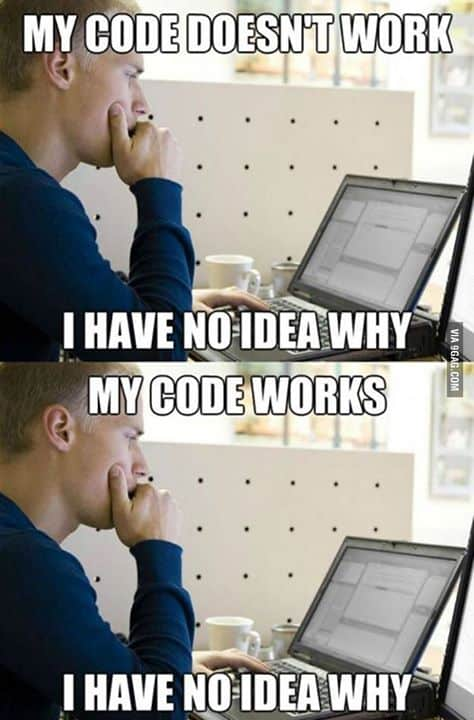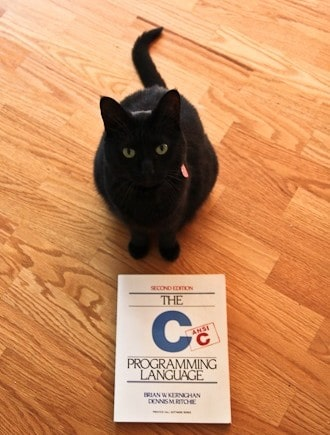# Comparison Pitfalls in C Programming LanguageYou probably at least heard something about C which is general purpose programming language. Speaking of coding in any programming language, there are some tricks which each programmer (especially beginner) should be aware of, otherwise he or she will be very surprised when analyzing the outcomes. In this article we’ll talk about such things as bit shift and type conversion in C.

The C programming language was created by Dennis Ritchie and Kenneth Thompson in 1969-1973 during their work in Bell Labs. It was an extension of the B language based on BCPL – one can understand why the C language is named so. If you think about language named A, you’re right: A Programming Language or APL was developed by Kenneth Iverson in 1964 for work with arrays, but that’s another story.

Nowadays, the pure C is widely used to learn programming and, among other more specific languages like AHDL, in the programming of microcontrollers present in many devices around us (cars, elevators etc.). And a lot of programs for PC are created using C++ which is extension of C to implement modern ideas and features of programming and similar languages like family .NET.

One can say that C is both very simple and hard simultaneously. It is simple because this language consists of a small number of statements. And it is hard due to many tricks which reduce the readability of the code: C makes it easy to shoot yourself in the foot; C++ makes it harder, but when you do it blows your whole leg off. (Bjarne Stroustrup)

If you are the beginner you need to be very attentive to details. Usually C programming homework assignments are designed to prevent students from hidden pitfalls. In this section we’re going to help you understand two important tricky features of C language.Each integer variable is saved in the computer memory as a sequence of bits, for example, one variable of int type needs $4$ bytes or $24$ bites (check info about different data types in C if needed). For example, the following code does not compare two values by a “<<” statement:
 int foo = 5, bar = 3; foo = foo << bar;
It shifts all the bits in the variable “foo” to the left by b positions. In our case $5$ is represented as $00000101$ in the memory and the operation shown in the code above produces the new value: $00101000$ (new three zeros appear at the right side) which is equal to $20$. As one can expect, $40=5\cdot 8=5\cdot 23$ and really, such bit shift is a fast way to multiply numbers by the powers of two! The shift by one position is equivalent to multiplication by $2$. And if one wants to shift bits to the right this is equivalent to a simple division by $2$. For example, we can divide $7$ by $2$ and obtain $3$ as follows:
 int foo = 7 >> 1;
Now foo equals $3$, because $\frac{7}{2^1} =\frac{7}{2}= 3.5$ and $3.5$ is rounded to $3$ during saving as integer variable. Another useful and important tool is type conversion. C language allows to use different types of variables, especially for numbers, such as float and double for real values; char and int for integer values. Also there are a few modifiers: short, long, signed and unsigned. The first two modifiers tell about the size of variable in memory (depending on platform, long types should not be shorter than regular ones). And the last two modifiers tell if the corresponding variable stores the sign of the number or not. For example, int foo or signed int foo (which is the same) can store numbers from $−2,147,483,648$ till $2,147,483,647$ and the unsigned int can store numbers from $0$ to $4,294,967,295$. The following code
 unsigned int i; for (i = N-1; i >= 0; --i) arr[i] = i;
will work infinitely, because when i equals $0$, the operation --i produces i equal to $4,294,967,295$.
One should be careful using numerical constants and variables of different types in arithmetical expressions, because in some cases the C compiler converts signed type variables to unsigned ones. Take a look at the following example:
 if (-1L << 1UL) printf("a"); if (-1L < 1UL) printf("b"); if (-1L == 1UL) printf("c"); if (-1L > 1UL) printf("d"); if (-1L >> 1UL) printf("e");
What will be printed into the prompt on a 32-bit machine for unambiguity? If you think that “b” will be included into the output, you’re wrong. The expression -1L tells compiler to create the negative numeric constant of long int type. And the expression 1UL tells it to create a positive numeric constant of the unsigned long int type. Each of the numerical constants -1L and 1UL is right, nevertheless the type conversion makes miracles with their comparison by the compiler. The standard of C demands the so-called integral promotion: converting all integers to the largest type of them and if this type is unsigned, all constants become unsigned. In the example above both constants become unsigned inside conditions of the each of the if-expressions. So, now we can say the output that one obtains.

1. Expression -1L << 1UL does not give zero anyway, because we multiply some huge positive number by $2$. The result is interpreted as “truth” and letter “a” is printed. We can say the same about the last “if” statement: the letter “e” will be printed.

2. Again, -1L becomes a huge positive number and it is larger than $1$, so the comparison is evaluated as “lie” and letter “b” is not printed. Also, because of that the letter “d” will be printed.

3. By the reason from the item above the left and the right side of comparison expression cannot be equal and letter “c” is not printed.

In conclusion, we obtain the sequence “ade” in the prompt.

Thus, be careful with this grandpa of modern programming languages and remember that more readable code is better code.0 Shares
Filed under Programming.